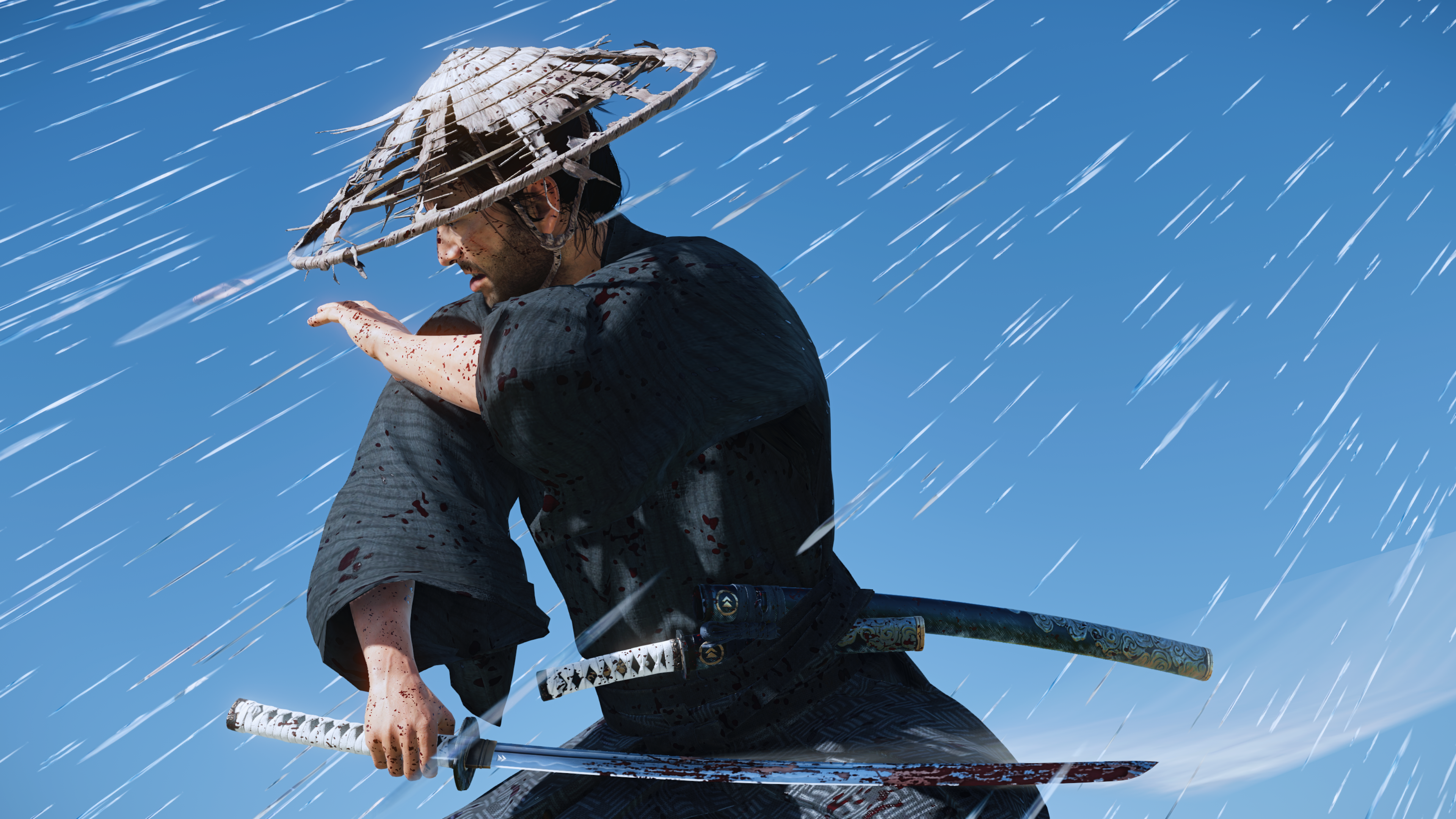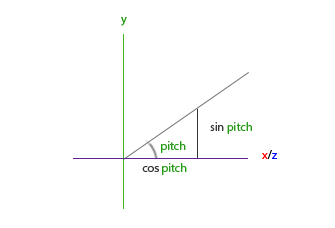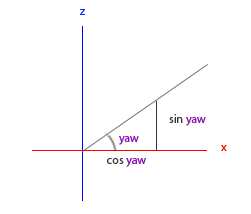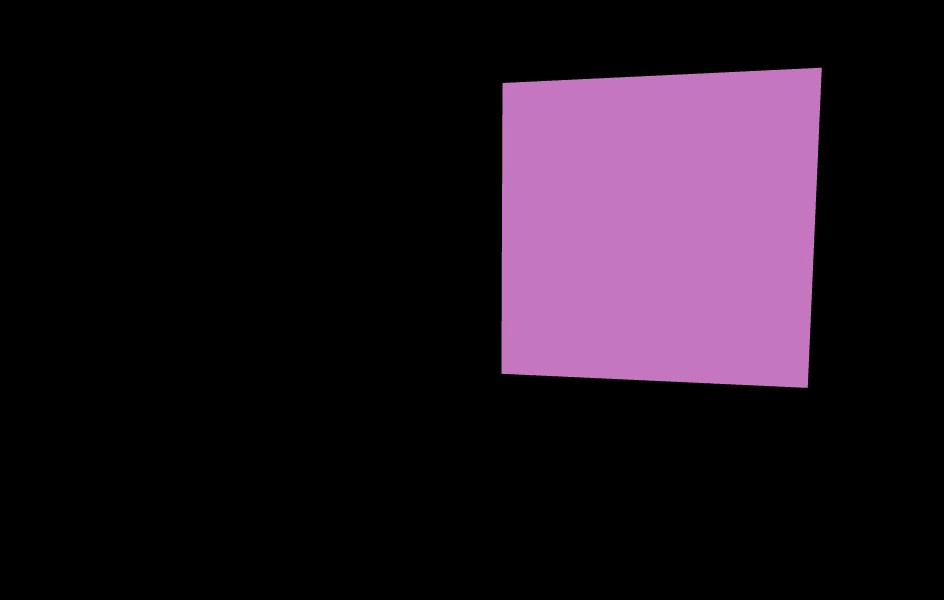Blog

Tutorial

2021年7月9日

# 摄像机

## 一.介绍对马岛之魂 镜井仁

• 移动坐标：前、后、左、右不同方向移动，一般移动都是通过键盘控制的，键位无所谓，你可以用w、a、s、d，也可以用↑、↓、←、→。
• 改变拍摄（视线）方向：一般游戏世界中改变拍摄方向都是通过鼠标的移动控制的，鼠标上移表示抬头的动作，下移表示低头，左移表示向左看，右移表示向右看。

## 二.类实现

3D图形学中的矩阵变换（二）文章中简单的介绍了摄像机的概念，并讲述了基变换lookAt矩阵。 摄像机的观察矩阵就是lookAt矩阵, 对矩阵证明感兴趣的可以去看一看我写的三篇有关矩阵证明的文章。

3d图形中的坐标系统文章中，我介绍了gl-matrix和glm, 这两个都是三维数学库，gl-matrix是js写的，glm是c写的，有了这两个库，我们就不需要自己手动去实现lookAt矩阵了。

gl-matrix和glm都提供了lookAt方法。函数的参数也完全相同，以gl-matrix为例，函数原型如下：

``function lookAt(out: mat4, eye: ReadonlyVec3, center: ReadonlyVec3, up: ReadonlyVec3): mat4;``
param description
mat4 存储结果矩阵。
eye 摄像机在世界坐标系中的坐标。
center 拍摄的视线中心。
up 世界坐标的上方向，一般为[0, 1, 0]。

``````var result = mat4.create();
mat4.lookAt(result, [0, 0, 10], [0, 0, 0], [0, 1, 0]);``````

``````class Camera {
public worldUp: vec3; // 世界方向的上方向向量
public position: vec3; // 摄像机位置
public front: vec3; // 摄像机的拍摄方向向量
}``````

``````class Camera {
...
public up: vec3; // 摄像机坐标系的上轴
public getViewMatrix() {
const center = vec3.add(vec3.create(), this.position, this.front);

return mat4.lookAt(mat4.create(), this.position, center, this.up); // 使用gl-matrix的lookAt方法获得lookAt矩阵
}
}``````

``````class Camera {
public worldUp: vec3; // 世界方向的上方向向量
public position: vec3; // 摄像机位置
public front: vec3 = [0, 0, -1]; // 摄像机的拍摄方向向量
public up: vec3 = [0, 1, 0]; // 摄像机坐标系的上轴，默认指向世界坐标系y轴正向
public right: vec3 = [1, 0, 0]; // 增加摄像机右轴，默认方向为世界坐标系x轴正向

public constructor(position: vec3 = [0, 0, 0], worldUp: vec3 = [0, 1, 0]) {
this.position = position;
this.worldUp = worldUp;

this.updateCameraVectors();
}

private updateCameraVectors() {
vec3.cross(this.right, this.front, this.worldUp); // 通过front和worldUp的向量叉积更新right
vec3.cross(this.up, this.right, this.front); // 通过right和front的叉积更新up向量
}

...
}``````

### 移动

``````/**移动方向 **/
enum Camera_Movement {
FORWARD,
BACKWARD,
LEFT,
RIGHT
}

class Camera {
...

public movementSpeed: number = SPEED; // 移动速度

public processKeyboard(direction: Camera_Movement, deltaTime: number) {
const velocity = this.movementSpeed * deltaTime;

switch (direction) {
case Camera_Movement.FORWARD:
var movement = vec3.scale(vec3.create(), this.front, velocity);
break;
case Camera_Movement.BACKWARD:
var movement = vec3.scale(vec3.create(), this.front, velocity);
vec3.sub(this.position, this.position, movement);
break;
case Camera_Movement.LEFT:
var movement = vec3.scale(vec3.create(), this.right, velocity);
vec3.sub(this.position, this.position, movement);
break;
case Camera_Movement.RIGHT:
var movement = vec3.scale(vec3.create(), this.right, velocity);
break;
default:
break;
}
}
}``````

`processKeyboard`方法比较简单，就是通过传入的移动方向枚举值，改变`position`变量。第二个参数`deltaTime`，是为了处理不同机器（性能不一样）可能帧频率不一样造成移动速度悬殊比较大的问题，所以开放一个参数用于调整。

### 视线方向

#### 俯仰角（pitch）``````const x = Math.cos(glMatrix.toRadian(pitch)); // 注意我们需要将pitch转化为弧度
vec3.normalize(this.front, [x, y, z]);``````

#### 偏航角``````const x = Math.cos(glMatrix.toRadian(yaw));
// y不受偏航角影响``````

``````const x = Math.cos(glMatrix.toRadian(yaw)) * Math.cos(glMatrix.toRadian(pitch));
vec3.normalize(this.front, [x, y, z]);``````

#### 更新Camera

``````const YAW = -90;
const PITCH = 0;
...

class Camera {
...

/**yaw angle: 偏航角 */
public yaw: number;
/**pitch angle: 俯仰角 */
public pitch: number;

public constructor(position: vec3 = [0, 0, 0], worldUp: vec3 = [0, 1, 0], yaw: number = YAW, pitch: number = PITCH) {
this.position = position;
this.worldUp = worldUp;
this.yaw = yaw;
this.pitch = pitch;

this.updateCameraVectors();
}

private updateCameraVectors() {
vec3.normalize(this.front, [x, y, z]);
vec3.cross(this.right, this.front, this.worldUp);
vec3.cross(this.up, this.right, this.front);
}
}``````

#### 监听鼠标移动

``````...
const SENSITIVITY = 0.1;

class Camera {
...

public mouseSensitivity: number = SENSITIVITY; // 鼠标灵敏度
public processMouseMovement(xoffset: number, yoffset: number, constrainPitch: boolean = true) {
xoffset *= this.mouseSensitivity;
yoffset *= this.mouseSensitivity;

this.yaw += xoffset;
this.pitch -= yoffset;
if (constrainPitch) {
if (this.pitch > 89) this.pitch = 89;
if (this.pitch < -89) this.pitch = -89;
}

this.updateCameraVectors();
}
}``````

### 缩放（附加功能）

``function perspective(out: mat4, fovy: number, aspect: number, near: number, far: number): mat4;``

``````const ZOOM = 45;
class Camera {
public zoom: number = ZOOM;

// 处理鼠标滚轮，控制zoom增减
public processMouseScroll(yoffset: number) {
this.zoom += yoffset;
if (this.zoom < 1) this.zoom = 1;
if (this.zoom > 60) this.zoom = 60;

this.updateCameraVectors();
}
}``````

``const projection = mat4.perspective(mat4.create(), glMatrix.toRadian(camera.zoom), gl.canvas.width / gl.canvas.height, 0.1, 100);``(完)